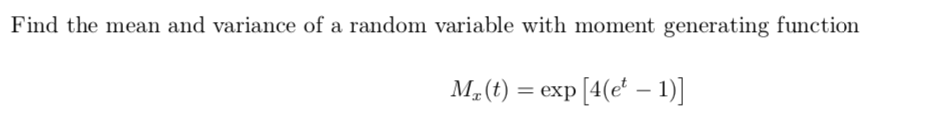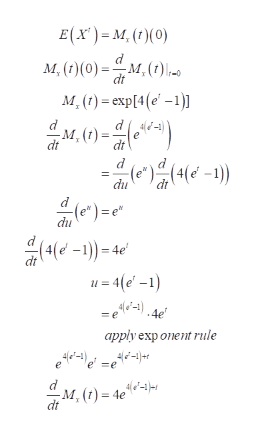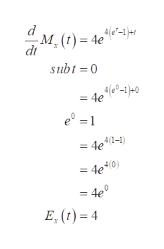# Find the mean and variance of a random variable with moment generating functionM2(t) = exp [4(e' – 1)]

Question
1 views

Question is attached.help_outlineImage TranscriptioncloseFind the mean and variance of a random variable with moment generating function M2(t) = exp [4(e' – 1)] fullscreen
check_circle

star
star
star
star
star
1 Rating
Step 1

Given that

The moment generating function of a random variable is

To find mean and variance:

Step 2

Mean:help_outlineImage TranscriptioncloseE(x') = M, (t)(0) M, (1)(0) = M, (?) .- dt M, (1) = exp[4(e' –1)] -M, dt (4(e' -1)) (e*). du (e*) =e" du (4(e -1)) = 4e' u = 4(e' -1) =e-).4e apply exp onent rule =e 4, (t) = 4e%o"-t}er dt fullscreen
Step 3

Now sub t=0 in differential equati...help_outlineImage TranscriptioncloseM. (t) = 4e%*-1}er dt subt =0 = 4e lo°-1}=0 e = 1 = de1-1) = 4el0) = 4e° E, (t) = 4 fullscreen

### Want to see the full answer?

See Solution

#### Want to see this answer and more?

Solutions are written by subject experts who are available 24/7. Questions are typically answered within 1 hour.*

See Solution
*Response times may vary by subject and question.
Tagged in

### Other